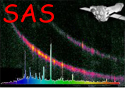XMM-Newton Science Analysis System

ommosaic (ommosaic-2.11) [xmmsas_20211130_0941-20.0.0]

### MAD test for data outliers

The MAD (median-absolute-deviation) test works as follows:
1. Compute the median value of the data values
2. For each data value compute its absolute deviation from the median
3. Compute the median of all the absolute deviations (the MAD value)
4. choose a value for nsigma
5. For each data value divide its computed deviation by the MAD value
6. If this value exceeds nsigma conclude that this is a deviant point

XMM-Newton SOC -- 2021-11-30